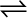7.21 Equilibrium constant, Kc for the reaction

N2 (g) + 3H2 (g)2NH3 (g) at 500 K is 0.061

At a particular time, the analysis shows that the composition of the reaction mixture is 3.0 mol L–1 N2, 2.0 mol L–1 H2, and 0.5 mol L–1 NH3. Is the reaction at equilibrium? If not in which direction does the reaction tend to proceed to reach equilibrium?

The given reaction is:

At a particular time

Now we know that,

It is given that ${K}_{c}=0.061.$

Since ${Q}_{c}\ne {K}_{c}$, the reaction is not an equilibrium.

Since ${Q}_{c}<{K}_{c}$, the reaction will proceed in the forward direction to reach equilibrium.